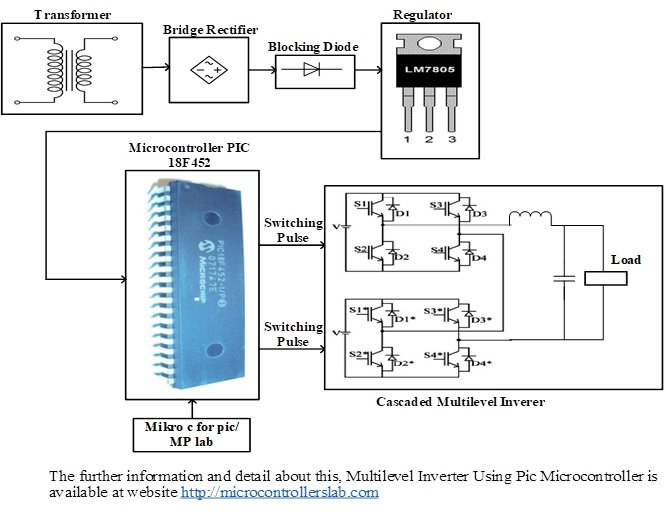# Multilevel inverter design Using Pic Microcontroller

Introduction to Multilevel Inverter Using Pic Microcontroller: Multilevel inverter using pic microcontroller is a such type of inverter that has been using for inverting the dc voltages into ac voltages. If we  take a small look on recently energy crises then we would clearly understand, that there is need to search the other energy resources to overcome the energy crises problem. So many renewable energy resources have developed such wind energy and solar energy etc. These energy resources have become so much popular because they are free of cost and environmentally friendly. But the energy, which is gained by these resources is dc energy therefore we cannot feed it directly into ac gird . This project is a simulation , design and implementation of multilevel inverter using PIC microcontroller. So now let’s start with applications of these type of inverters.

### Applications of multilevel inverter using pic microcontroller

So renewable energy is firstly inverted into ac then it is fed into ac power gird. This energy conversion process is done with the help of power electronic technology. So multilevel inverter is a simple example of power electronic technology . So many dc to ac power electronic inverters are available in market but they do not provide pure sine wave as well as  they are not so much efficient and reliable. Besides this, they are  so much costly . Here we have designed a power electronics inverter that is a called multilevel inverter using pic microcontroller. This multilevel inverter has designed with the help power electronics switches such as MOSET or IGBTs ,step down transformer, bridge rectifier, voltage regulator, pic18F452 microcontroller, and filter circuit etc. This multilevel inverter is less costly, so much efficient, reliable and provides sine wave with low harmonic content. The block diagram of this multilevel inverter using pic microcontroller with all their essential component is shown in figure 1

### Block Diagram of Multilevel Inverter Using Pic Microcontroller

Here is the block diagram of multilevel inverter using pic microcontroller with all their essential component is,Figure 1 Block Diagram of Multilevel Inverter Using Pic Microcontroller with all their Essential Components

### Working Principle of Multilevel Inverter Using Pic Microcontroller

This multilevel inverter using pic microcontroller works on the principle of PWM (pulse width modulation) technique. The PWM wave is generated by the pic microcontroller for turning on or off the multilevel inverter switches. Microcontroller is the main intelligent controller of this multilevel inverter. It is programmed in c language with the help of mikro/c software. It is powered up with 5 volts dc voltages which are gained from voltage regulator after stepping down and rectifying the ac voltages shown in block diagram. For obtaining five level inverter outputs such as Vo= Vdc ,V0=Vdc/2 , Vo= 0, V0=-Vdc/2  and Vo= -Vdc  two H bridge inverters are connected in cascaded form shown in block diagram .

This cascaded multilevel inverter consists of eight switches such S1S1,S2S2,S3S3 and S4S4. These switches are turned on or off through microcontroller programming. In first cycle, four switches such S1S1and S4S4 are turned on simultaneously then output voltages would be equal to dc voltages means Vo= Vdc . In second cycle, S1S4 would be turned on but remaining switches would be turned off then output voltages would be equal to V0=Vdc/2 . In third cycle all switches would be turned off  simultaneously then output voltages would be equal to Vo= 0. In fourth cycle,S2S3 switches would be turned on then output voltages would be equal toV0=-Vdc/2 . Similarly, in fifth cycle, four switches such as S2S2 and S3S3 would be turned on simultaneously then output voltages would be equal to Vo= -Vdc . So the fifth level inverter work on these principles. Filter circuit which consist of capacitor and inductor is also connected this multilevel inverter for reducing the total harmonic distortion (THD) content.

### 2 thoughts on “Multilevel inverter design Using Pic Microcontroller”

1. sir,i liked your projects and i have a question for this one
how to visualize the ac voltage across the load ???